Rigid Transformations - Isometries MathBitsNotebook.com Terms of Use   Contact Person: Donna Roberts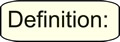A rigid transformation (also called an isometry) is a transformation of the plane that preserves length. Reflections, translations, rotations, and combinations of these three transformations are "rigid transformations".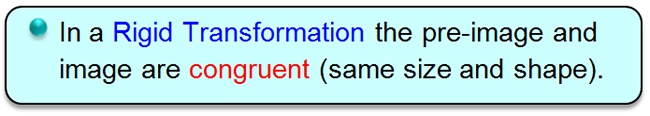While the pre-image and the image under a rigid transformation will be congruent,
they may not be facing in the same direction.Reflection in a Line
For a review of reflections, see the Refresher section Transformations: Reflections.
Now let's expand that knowledge of reflections in relation to geometry.

 A reflection over line m (notation rm ) is a transformation in which each point of the original figure (pre-image) has an image that is the same distance from the reflection line as the original point, but is on the opposite side of the line. A reflection is called a rigid transformation or isometry because the image is the same size and shape as the pre-image.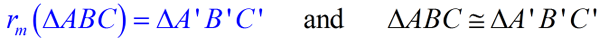The reflection line, m, is the perpendicular bisector of the segments joining each point to its image. Notice that these segments are parallel, since they are perpendicular to the same line.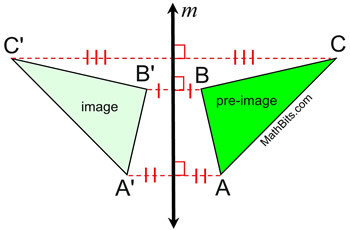In this reflection that maps ΔABC to ΔA'B'C', the distances from the pre-image points to the image points vary (are not necessarily equal), but the segments representing these distances are parallel.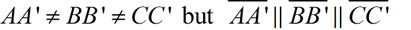Orientation (lettering): The lettering of the points of the pre-image, in this diagram, is clockwise A-B-C, while the image is lettered counterclockwise A'-B'-C'. When lettering changes direction, in this manner, the transformation is referred to as a non-direct or opposite isometry.
 Properties preserved under a line reflection from the pre-image to the image. 1. distance (lengths of segments remain the same) 2. angle measures (remain the same) 3. parallelism (parallel lines remain parallel) 4. collinearity (points remain on the same lines) ---------------------------------------------------------- The orientation (lettering around the outside of the figure), is not preserved. The order of the lettering in a reflection is reversed (from clockwise to counterclockwise or vice versa).
Common Reflections: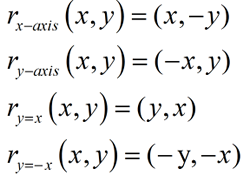See these reflections, and others,
as "examples" on the page
Transformations: Reflections.

When formally defining a reflection, there are two situations (cases) that need to be considered for a precise definition. Is the point being reflected lying ON the line of reflection, or NOT ON the line of reflection?A reflection is a rigid transformation (isometry) that maps every point P in the plane to point P', across a line of reflection, m, such that:
 Case 1: if point P is ON line m, the point is its own reflection (P = P') and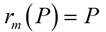(point P is "fixed").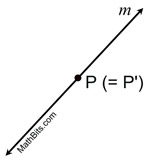Case 2: if point P is NOT on line m, then m is the perpendicular bisector of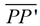where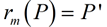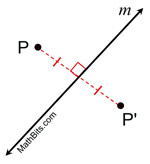Translations
For a review of translations, see the Refresher section Transformations: Translations.
Now let's expand that knowledge of translations in relation to geometry.

 A translation (notation Ta,b ) is a transformation which "slides" a figure a fixed distance in a given direction. In a translation, ALL of the points move the same distance in the same direction. A translation is called a rigid transformation or isometry because the image is the same size and shape as the pre-image.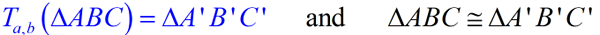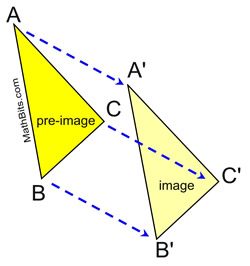In this translation that maps ΔABC to ΔA'B'C', the distances from the pre-image points to the image points are equal, and the segments representing these distances are parallel.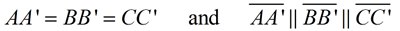In addition, the corresponding segment sides of the pre-image and image are parallel.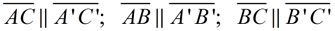Orientation (lettering): The lettering of the points of the pre-image, in this diagram, is counterclockwise A-B-C, and the image is also lettered counterclockwise A'-B'-C'. When lettering order remains the same, the transformation is referred to as a direct isometry.
 Properties preserved under a translation from the pre-image to the image. 1. distance (lengths of segments remain the same) 2. angle measures (remain the same) 3. parallelism (parallel lines remain parallel) 4. collinearity (points remain on the same lines) 5. orientation (lettering order remains the same)
Mapping notation:
(x, y) → (x+a, y+b)

See translations "examples" on the page
Transformations: Translations.A translation is a rigid transformation of the plane that moves every point of a pre-image a constant distance in a specified direction.

There are several ways to indicate that a translation is to occur:

 mapping: Example:     (x, y) → (x + 8, y - 6) Read: "the x and y coordinates are mapped to x + 8 and y - 6 respectively". notations: Example:    T8,-6    or T8,-6 (x, y) = (x + 8, y - 6) The 8 tells you to "add 8" to all x-coordinates, while the -6 tells you to "subtract 6" from all of the y-coordinates. description: Example: "8 units to the right and 6 units down." A verbal or written description of the translation is given. Movement: Remember that adding a negative value (subtracting), indicates movement left and/or down, while adding a positive value indicates movement right and/or up. Using vectors to show movement of a translation: A vector is represented by a directed line segment, a segment with an arrow at one end indicating the direction of movement. Unlike a ray, a directed line segment has a specific length. The Pythagorean Theorem can be used to find the length of a vector in the coordinate plane. Vectors used in translations are what are known as "free vectors", which are a set of parallel directed line segments. A vector translation moving "8 units to the right and 6 units down" can be written as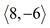. You may also see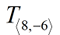.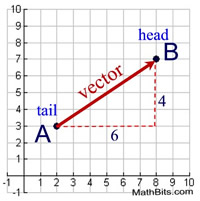Read more about vectors.

There is an "identity" translational transformation that maps all points onto themselves (no movement of the pre-image), expressed as (x, y) → (x, y). (You may see the identity transformation denoted by the letter I.)Rotations
For a review of rotations, see the Refresher section Transformations: Rotations.
Now let's expand that knowledge of rotations in relation to geometry.

A rotation of θ degrees (notation RC,θ ) is a transformation which "turns" a figure about a fixed point, C, called the center of rotation. When working in the coordinate plane, the center of rotation should be stated, and not assumed to be at the origin. Rays drawn from the center of rotation to a point and its image form an angle called the angle of rotation. A rotation is called a rigid transformation or isometry because the image is the same size and shape as the pre-image.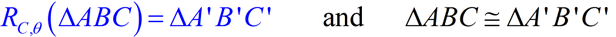An object and its rotation are the same shape and size, but the figures may be positioned differently.

During a rotation, every point is moved the exact same degree arc along the circle
defined by the center of the rotation and the angle of rotation.
(The dashed arcs in the diagram below represent the circles, with center P, through each of the triangle's vertices.
Note that PC=PC', for example, since they are the radii of the same circle.)

A positive angle of rotation turns a figure counterclockwise (CCW),
and a
negative angle of rotation turns the figure clockwise, (CW).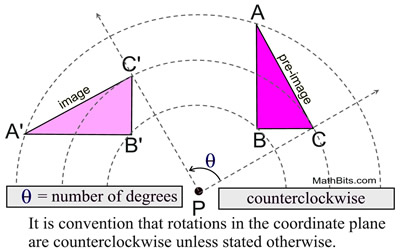In this rotation that maps ΔABC to ΔA'B'C', the distances from the pre-image points to the image points vary (as they are the radii of the circles). Unlike reflections and translations, the segments connecting pre-image and image points are NOT parallel. Orientation (lettering): The lettering of the points of the pre-image, in this diagram, is counterclockwise A-B-C, and the image is also lettered counterclockwise A'-B'-C'.
 Properties preserved under a rotation from the pre-image to the image. 1. distance (lengths of segments remain the same) 2. angle measures (remain the same) 3. parallelism (parallel lines remain parallel) 4. collinearity (points remain on the same lines) 5. orientation (lettering order remains the same)
Common Graph Rotations:
(center at the origin, O)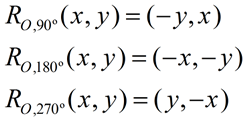See rotation "examples" on the page
Transformations: Rotations.
 There are two possible directions to travel when rotating. Look at point P in the diagram at the right. The positive x-axis is considered the "starting" location of 0º. You can have an angle of rotation of positive 135º counterclockwise to point P, or a negative angle of 225º clockwise to point P. Notice that together, the angles (135º and 225º) form a complete circle (360º). It is even possible to have one complete revolution (360º) PLUS and additional 135º to get to P (an angle of rotation of 495º).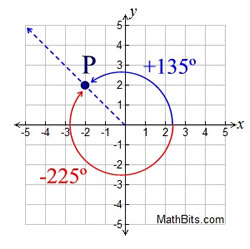When working in the coordinate plane, it is important to have a visual understanding of how the quadrants are divided through rotational angles. The most common angles seen on the grid are multiples of 15º. The "favorite" angles are usually 30º, 45º, 60º, 90º, 180º, and 270º.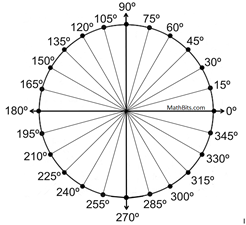There is an "identity" rotational transformation of 0º that maps all points onto themselves (no movement of the pre-image), written RC,0 (P) = P. (You may also see the identity transformation denoted by the letter I.)